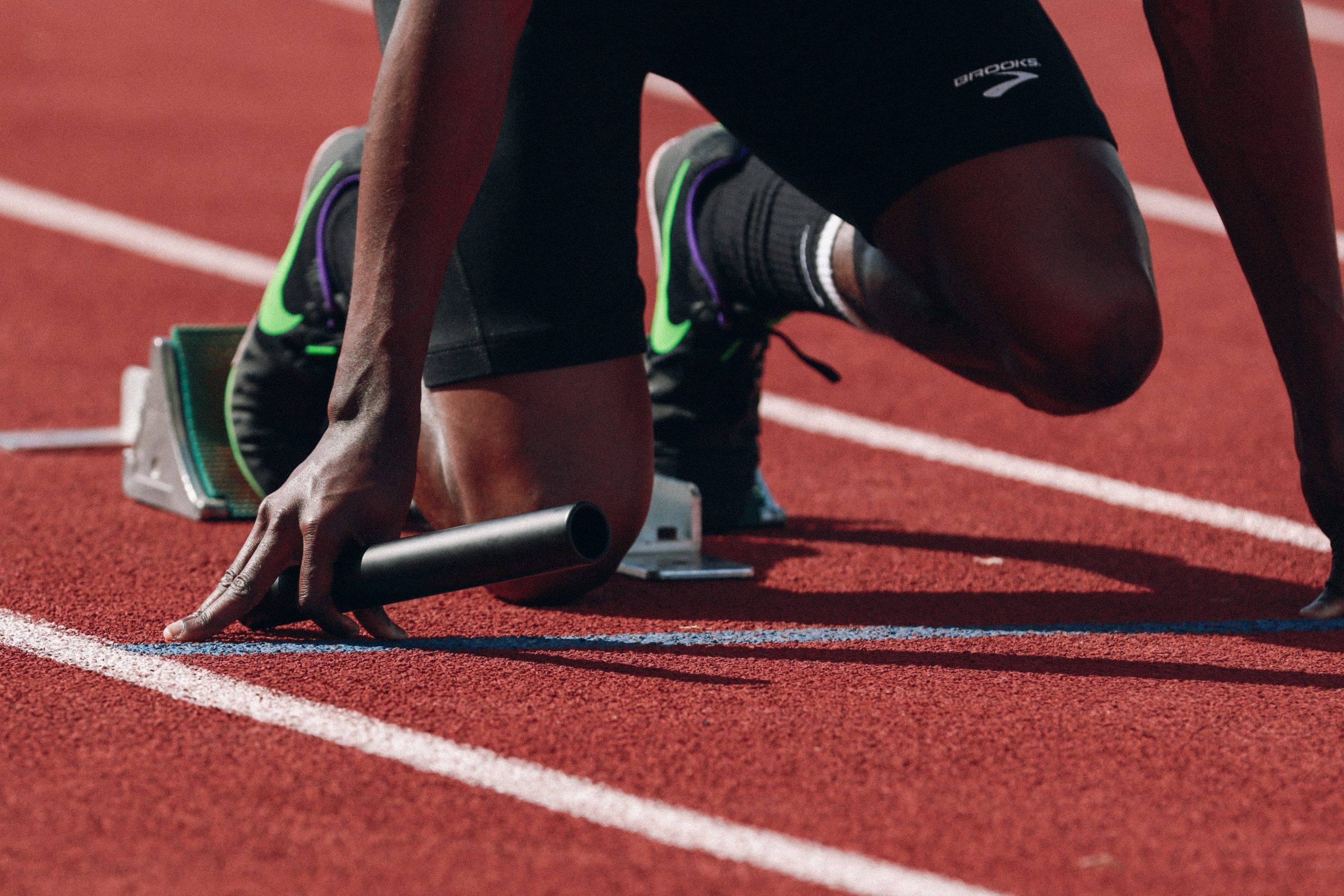# 17 3.0 IntroductionFigure 1. The results of many track and field events are the outcome of kinematic variables. Runners will try to cover the distance in the shortest time possible whereas jumpers will attempt to cover a greater displacement than their opponent.

Movement is everywhere we look. When we approach the topic of human movement, we can consider the movement of the body itself (walking, jumping etc..) or of object’s that move as a consequence of human motion (tennis ball, hockey stick etc..). Questions about motion are interesting in and of themselves: How long will it take for me to walk to work this morning? Where will a football land if it is thrown at a certain angle? But an understanding of motion is also key to understanding other concepts in biomechanics. An understanding of acceleration, for example, is crucial to the study of force.

Our formal study of biomechanics begins with kinematics which is defined as the study of motion without considering its causes. The word “kinematics” comes from a Greek term meaning motion and is related to other English words such as “cinema” (movies) and “kinesiology” (the study of human motion).

Movement can be separated into two main types: Linear and Angular. Linear motion refers to motion of a body along a straight or curved line. Angular motion refers to the movement of a body about a fixed axis. Let’s begin with the analysis of linear movement.

In this chapter, we examine the simplest type of motion—namely, motion along a straight line, or one-dimensional motion. In following chapters, we apply concepts developed here to study motion along curved paths (two-dimensional motion); for example, that of a bicycle rounding a curve.